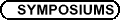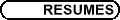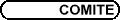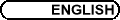Algebraic Combinatorics / Combinatoire algébriqueOrg: François Bergeron, Riccardo Biagioli, Peter McNamara and/et Christophe Reutenauer (UQAM) NANTEL BERGERON, York University Hopf algebra and representation: the Hecke-Clifford case The Grothendieck ring of a Tower of algebras is equipped with a graded Hopf algebra structure. We have many examples of this phenomena where we represent interesting graded Hopf algebras. Here we provide a representation theoretical interpretation of the peak algebra and its graded dual as Grothendieck rings of the tower of Hecke-Clifford algebras at q=0. FRANCESCO BRENTI, Università di Roma II, Via della Ricerca Scientifica 1, 00133 Roma, Italy Combinatorics of parabolic Kazhdan-Lusztig and R-polynomials for Hermitian symmetric pairs The parabolic Kazhdan-Lusztig and R-polynomials were introduced by Deodhar in 1987, and are defined for any two elements of any quotient of any Coxeter group. They include the classical Kazhdan-Lusztig and R-polynomials, which correspond to elements of the groups themselves, and have applications to representation theory and geometry. Hermitian symmetric pairs are quotients of Weyl groups with particularly nice geometric properties. Combinatorially, these are quotients that, as posets under Bruhat order, are distributive lattices. It is therefore natural to wonder if the parabolic Kazhdan-Lusztig and R-polynomials, which are usually very difficult to compute, also have particularly nice properties in the Hermitian symmetric case. The purpose of this talk is to show that this is indeed the case. More precisely, we show that for Hermitian symmetric pairs the R-polynomials are always given by a nice product formula, and that the Kazhdan-Lusztig ones are always a monomial. In the case of classical Hermitian symmetric pairs we show how one can compute combinatorially all the exponents appearing in a given polynomial from the representation of the elements of these quotients as (possibly signed) permutations and (possibly shifted) partitions. In particular, for the Kazhdan-Lusztig ones, this involves a generalization of the concept of Dyck partition (introduced in [F. Brenti, Pacific J. Math., 207(2002), 257-286] for the computation of these polynomials in type A) to shifted partitions. We also show that the polynomials are combinatorial invariants. Namely that they depend only on the interval determined by the defining elements under Bruhat order as an abstract poset, and not on the elements themselves, or on the particular Hermitian symmetric pair. SERGEY FOMIN, University of Michigan, Ann Arbor, MI 48109, USA Catalan combinatorics of arbitrary type The Catalan numbers and their q-analogues involving Narayana numbers have natural counterparts for an arbitrary finite Coxeter group. These numbers come up in a variety of combinatorial contexts, some of which will be surveyed in the talk. IAN GOULDEN, University of Waterloo, Waterloo, Ontario N2L 3G1 A direct bijection for the Harer-Zagier formula We give a combinatorial proof of Harer and Zagier's formula for the disjoint cycle distribution of a long cycle multiplied by an involution with no fixed points, in the symmetric group on a set of even cardinality. The main result is a direct bijection for a set Bp,k, the enumeration of which is equivalent to the Harer-Zagier formula. The elements of Bp,k are of the form (m,p), where m is a pairing on {1,...,2p}, p is a partition into k blocks of the same set, and a certain relation holds between m and p. (The set partitions p that can appear in Bp,k are called "shift-symmetric", for reasons that are explained in the talk.) The direct bijection for Bp,k identifies it with a set of objects of the form (r,t), where r is a pairing on a 2(p-k+1)-subset of {1,...,2p} (a "partial pairing"), and t is an ordered tree with k vertices. If we specialize to the extreme case when p=k-1, then r is empty, and our bijection reduces to a well-known tree bijection. This is joint work with Alexandru Nica. FREDERICO INCITTI, KTH, Stockholm DAVID JACKSON, University of Waterloo, Waterloo, Ontario Combinatorial aspects of the Temperley-Lieb algebra The Temperley-Lieb algebra TLn is an instance of an algebra whose generators may be represented by planar diagrams of great simplicity. We show that an enrichment of TLn may be used to provide a very natural proof of non-singularity of the matrix of chromatic joins. This matrix was introduced by Tutte in the course of his long preoccupation with the algebraic approach proposed by Birkhoff-Lewis in the 1940s to the Four Colour Problem, and he gave the first proof of its non-singularity. TLn can be regarded as a subalgebra of the partition algebra, and there are a number of interesting consequences of this view. The first is that there is an orthogonal projection from the partition algebra to TLn that has a very natural interpretation in terms of the Birkhoff-Lewis approach. The second is a nice connexion with the diagrammatics of topological quantum field theory and with Schur-Weyl duality. The latter comments relate to work in progress and the details and further implications are yet to be completed, so I shall touch upon them only briefly. This is joint work with Sabin Cautis. MERCEDES ROSAS, York University Noncommutative invariants and coinvariants of the symmetric group We will present a noncommutative version of the classical theory of invariants and coinvariants of the symmetric group, as well as a noncommutative version of a theorem of Chevalley that says that the ring of polynomials can be written as the tensor product of its invariants times its coinvariants. A brief review of the clasical theory of invariants and coinvariants of the symmetric group will be included in this talk. This is joint work with Bruce Sagan, Nantel Bergeron, Christophe Reutenauer, and Michael Zabrocki. MARK SKANDERA, Dartmouth College Schur nonnegative polynomials We define a symmetric function to be Schur nonnegative (SNN) if it is equal to a nonnegative linear combination of Schur functions. We define a polynomial p(x1,1,...,xn,n) in n2 variables to be Schur nonnegative if for every Jacobi-Trudi matrix A = (ai,j), the symmetric function p(a1,1,...,an,n) is SNN. Using the Kazhdan-Lusztig basis for C[Sn], we will show that certain differences of products of matrix minors are SNN polynomials. From these we will deduce that certain differences of products of Schur functions are SNN symmetric functions. RICHARD STANLEY, M.I.T., Cambridge, MA 02139, USA Crossings, nestings, oscillating tableaux, and vacillating tableaux It is well-known that the number of complete matchings (or partitions into n blocks of size 2) of the set {1,2,...,2n} with no crossings or with no nestings is the Catalan number Cn. We discuss how the theory of oscillating tableaux, which originally arose in the representation theory of the symplectic and orthogonal groups and the Brauer algebra, can be used to obtain further results on crossings and nestings of matchings. In particular, if fn(i,j) is the number of complete matchings on {1,2,...,2n} with at most i mutually crossing edges and with at most j mutually nested edges, then fn(i,j) = fn(j,i). Moreover, the Gessel-Zeilberger reflection principle allows the determination of the generating function Fi(x) = ån ³ 0 fn(i,¥) [(x2n)/((2n)!)]. Similar results hold when matchings are replaced with set partitions, using a variation of oscillating tableaux called vacillating tableaux. This is joint work with William Y. C. Chen, Eva Y. P. Deng, Rosena R. X. Du, and Catherine H. Yan. JOHN STEMBRIDGE, University of Michigan, Ann Arbor MI 48109-1109, USA Graded multiplicities in the Macdonald kernel and a bi-graded co-invariant algebra For each root system, there is a Macdonald kernel-it is the symmetric bilinear form relative to which the Macdonald polynomials are orthogonal. It may also be viewed as a virtual character with coefficients that are formal power series in q and t. Various specializations of it are connected to classical work of Kostant and Chevalley. For example, when q=0, the graded multiplicities of the irreducible characters are (up to normalization) polynomials in t with nonnegative coefficients. Aside from the type A case, the combinatorics of these polynomials still remain rather mysterious. In this talk, I will describe a general method for decomposing the Macdonald kernel into irreducible characters, and some of the consequences of this decomposition (e.g., explicit formulas, some proved, some conjectured). The most interesting result relates the q,t-multiplicities in the Macdonald kernel with a bi-graded generalization of the co-invariant algebra of the associated Weyl group. This generalizes work of A. Broer (1995), Y. Bazlov (2001), and J. Stembridge (Ph.D. thesis, 1985), and both proves and generalizes a conjecture of M. Reeder (1997). STEPHANIE VAN WILLIGENBURG, University of British Columbia Decomposable compositions and equality of ribbon Schur functions In the Hopf algebra of noncommutative symmetric functions the set of ribbon Schur functions forms a basis, however, in the Hopf algebra of symmetric functions the commutative image of the ribbon Schur functions only forms a spanning set. This raises the question: what are the relations that arise amongst the commutative ribbon Schur functions? In particular, when are two equal? In this talk we define an equivalence relation on integer compositions such that two commutative ribbon Schur functions, indexed by compositions, are equal if and only if their indices are equivalent in this sense. As an application we derive identities on certain Littlewood-Richardson coefficients. MIKE ZABROCKI, University of York Pattern avoidance and regular languages The problem of enumerating classes of permutation which avoid certain patterns is not new to algebraic combinatorics but very few general techniques exist for attacking this problem. I will present a proof that permutations which avoid any given pattern and have a fixed number of descents are regular and show how this can be used to generalize the standard notion of pattern avoidance and containment. This will also give a procedure for determining the generating function for patterns which avoid a pattern and have a fixed number of descents. This is joint work with Andrew Rechnitzer and Murray Elder.